﻿ Further Improvement of Simpson-type Methods for Solving Nonlinear EquationsAmerican Journal of Computational and Applied Mathematics

p-ISSN: 2165-8935    e-ISSN: 2165-8943

2019;  9(3): 57-61

doi:10.5923/j.ajcam.20190903.02### Further Improvement of Simpson-type Methods for Solving Nonlinear Equations

R. Thukral

Padé Research Centre, 39 Deanswood Hill, Leeds, West Yorkshire, LS17 5JS, England

Correspondence to: R. Thukral, Padé Research Centre, 39 Deanswood Hill, Leeds, West Yorkshire, LS17 5JS, England.
 Email: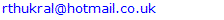Abstract

The aim of this paper is to present further improvement of the Simpson-type methods for finding zeros of a nonlinear equation. The new Simpson-type methods are shown to converge of the same order four and five, but with better precision of the zeros. In terms of computational cost, the new iterative methods require four evaluations of functions per iteration and therefore the new methods have an efficiency index better than the classical Simpson method. It is proved that the new methods have a convergence of order four and five. We examine the effectiveness of the new Simpson-type methods by approximating the simple root of a given nonlinear equation. Numerical comparisons are included to demonstrate exceptional convergence speed of the proposed methods and thus verifies the theoretical results.

Keywords: Simpson-type methods, Newton method, Simple root, Nonlinear equation, Root-finding, Order of convergence

Cite this paper: R. Thukral, Further Improvement of Simpson-type Methods for Solving Nonlinear Equations, American Journal of Computational and Applied Mathematics , Vol. 9 No. 3, 2019, pp. 57-61. doi: 10.5923/j.ajcam.20190903.02.

### 1. Introduction

In this paper, we present an alternative new fourth and fifth-order iterative methods to find a simple root of the nonlinear equation. It is well known that the techniques to solve nonlinear equations have many applications in Mathematics and applied science. Two well-known techniques, namely the classical Newton method and the classical Simpson method, for their simplicity with convergence order of two and three respectively [1-14], are applied to construct the new Simpson-type methods. It already has been established that the new Simpson-type method requires same amount of evaluations of the function as the classical Simpson method and it has been established that the new Simpson-type iterative methods have a better efficiency index than the classical Simpson method [11-13]. The prime motive for the development of the new Simpson-type methods was to establish an alternative scheme and demonstrate exceptional convergence speed of the proposed methods.
The remaining sections of the paper are organized as follows. Some basic definitions and construction of the new Simpson-type methods are discussed in section 2. In section 3, we prove the order of convergence of the new Simpson-type methods. Finally, in section 4, numerical comparisons are made to demonstrate the performance of the presented methods.

### 2. Construction of the New Iterative Methods

#### 2.1. Preliminaries

In order to establish the order of convergence of the new Simpson-type methods iterative method, we use the following definitions [3, 9, 11, 14].
Definition 1 Let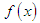be a real-valued function with a root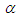and let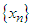be a sequence of real numbers that converge towards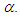The order of convergence p is given by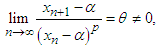(1)
where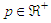and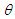is the asymptotic error constant.
Definition 2 Let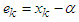be the error in the k-th iteration, then the relation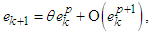(2)
is the error equation. If the error equation exists, then p is the order of convergence of the iterative method.
Definition 3 Let r be the number of function evaluations of the method. The efficiency of the method is measured by the concept of efficiency index and defined as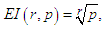(3)
where p is the order of convergence of the method .
Definition 4 Suppose that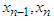and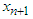are three successive iterations closer to the root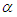of (1). Then the computational order of convergence may be approximated by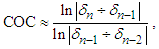(4)
where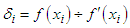.
Before we define the new fourth and fifth-order Simpson-type methods, we state essentially the classical third-order Simpson method and the recently introduced fourth and fifth-order Simpson-type methods [12, 13].

#### 2.2. The Classical Simpson Third-order Method

Since this method is well established [1, 2, 4-6, 8, 10-13], we shall state the essential expressions used in order to calculate the root of the given nonlinear equation. Hence the Simpson third-order method is given as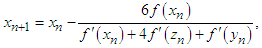(5)
where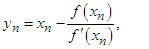(6)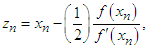(7)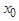is the initial approximation and provided that the denominator of (5) is not equal to zero.

#### 2.3. The Simpson-type Fourth-order Methods

In this section we state the recently introduced fourth-order Simpson-type method  to find simple root of a nonlinear equation. The improvement of the classical Simpson method was made by introducing a new factor in (5). Hence, the improved fourth-order Simpson-type method is given by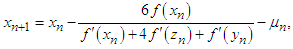(8)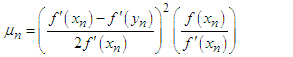(9)
This is actually a simplified version of fourth-order Simpson-type method given in .

#### 2.4. New Simpson-type Fourth-order Methods

In this section we construct an alternative fourth-order Simpson-type method for finding simple root of a nonlinear equation. The improvement of the classical Simpson method was made by introducing a new factor in denominator of (5). Hence, the improved fourth-order Simpson-type method is given by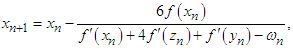(10)
where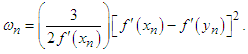(11)

#### 2.5. The Simpson-type Fifth-order Method

The fifth-order Simpson-type method for finding simple root of a nonlinear equation has been presented in . The order of convergence of the classical Simpson method and the fourth-order Simpson-type method is increased by introducing two different type of factors in (5). Hence, the fifth-order Simpson-type method is given by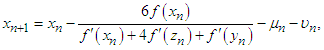(12)
where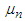is given by (9) and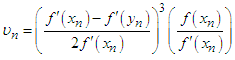(13)
Here also we present a simplified version of fifth-order Simpson-type method given in .

#### 2.6. New Simpson-type Fifth-order Method

Here we present an alternative to fifth-order Simpson-type method (12) for finding simple root of a nonlinear equation. Here also, we improve the performance of (12) by introducing two new different type of factors in the denominator of (5). Hence, the new fifth-order Simpson-type method is given by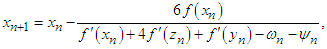(14)
where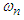is given by (11) and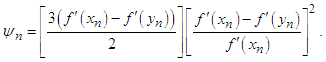(15)

### 3. Convergence Analysis

In this section, we prove the order of convergence of the new fourth and fifth-order Simpson-type methods, given by (10) and (14) respectively.
Theorem 1
Let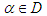be a simple zero of a sufficiently differentiable function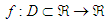for an open interval D. If the initial guess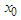is sufficiently close to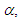then the order of convergence of the new Simpson-type methods defined by (10) and (14) is four and five, respectively.
Proof
Let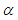be a simple root of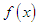, i.e.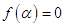and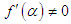, and the error is expressed as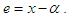(16)
Using the Taylor series expansion and taking into account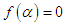, we have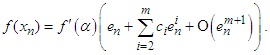(17)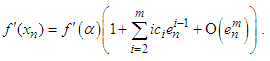(18)
where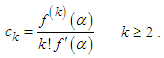(19)
Dividing (17) by (18), we get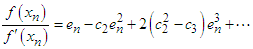(20)
and hence, we have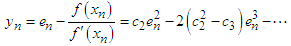(21)
The expansion of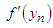about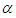is given as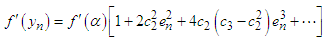(22)
Since from (7) we have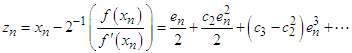(23)
Taylor expansion of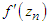about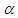is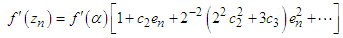(24)
The denominator of (5) is given as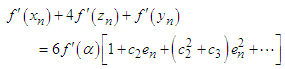(25)
Dividing the numerator by denominator of (5), we get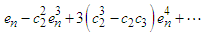(26)
It is well established that the error equation of (5) is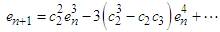(27)
The improvement factor introduced in fourth-order method (8) is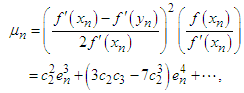(28)
and the error equation yields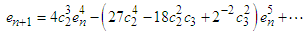(29)
Furthermore, the factor introduced in the fifth-order (12) is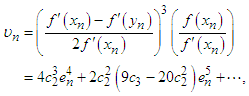(30)
and the error equation yields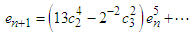(31)
For the purpose of this paper, a new different factor is introduced in denominator of (5) and a new fourth-order Simpson-type method is obtained (10). The new factor is given as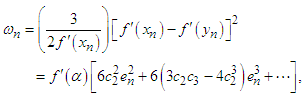(32)
and the error equation yields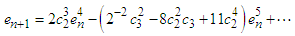(33)
The new fourth-order Simpson-type method is improved by introducing another factor in (10) and we obtain a new fifth-order Simpson-type method (14). The new factor is given by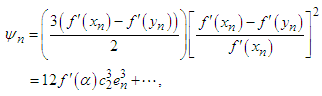(34)
and the error equation yields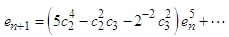(35)
The error equations (33) and (35) establishes the new fourth-order Simpson-type method and the new fifth-order Simpson-type method defined by (10) and (14) respectively.

### 4. Application of the New Simpson-type Iterative Methods

In this section, numerical results on some test functions are compared for the new Simpson-type methods (10) and (14) with the established Simpson-type methods. It is apparent that the efficiency index of the new methods (10) and (14) is similar to (8) and (12) respectively. The efficiency index is calculated by the formula (3). Hence, the efficiency index of the new Simpson-type methods given by (14) and (12) is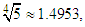whereas the efficiency index of the fourth-order Simpson-type methods given by (10) and (8) is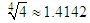and the efficiency index of the classical Simpson third-order method is given by (5) is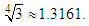To demonstrate the performance of the new iterative methods, we display the difference between the simple root and the approximation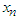for test functions with initial guess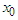. In fact,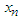is calculated by using the same total number of function evaluations for all Simpson-type methods.
Numerical example 1
We will demonstrate the performance of the Simpson-type methods for the following nonlinear equation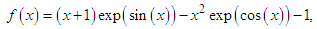(36)
the exact value of the simple root of (36) is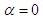In Table 1 the errors obtained by the Simpson-type methods described, is based on the initial value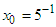. We observe that all the Simpson-type methods are converging to the expected order.
 Table 1. Errors occurring in the estimates of the root of (36) by the methods described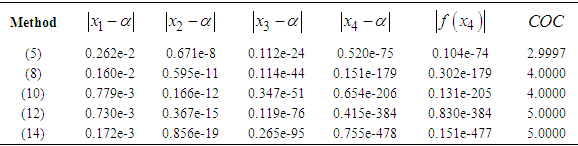Numerical example 2
We will demonstrate the performance of the Simpson-type methods for the following nonlinear equation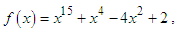(37)
the exact value of the simple root of (37) is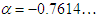In Table 2 the errors obtained by the Simpson-type methods described, is based on the initial value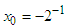. We observe that the new Simpson-type methods are converging to the expected order.
 Table 2. Errors occurring in the estimates of the root of (37) by the methods described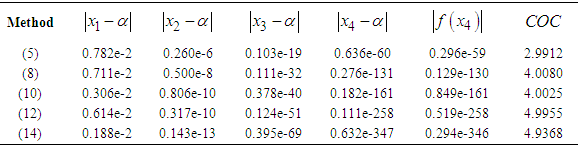### 5. Remarks and Conclusions

In this paper, we have demonstrated the performance of the new Simpson-type methods, namely the Simpson-type fifth-order method and the Simpson-type fourth-order method. The prime motive of the development of the new Simpson-type methods was to establish an alternative iterative method than the classical Simpson third-order iterative method and recently introduced the Simpson-type fourth and fifth-order iterative methods. We have examined the effectiveness of the new Simpson-type methods by showing the accuracy of the simple root of a nonlinear equation. It is observed from tables that the proposed methods (10) and (14) have a better performance as compared with recently introduced Simpson-type methods given (8) and (12) respectively. Also, it is well-established that the efficiency index of the new fifth-order methods is much better than the fourth-order Simpson-type methods and the classical Simpson third-order method. Numerical comparisons are made to demonstrate the performance of the derived method. Finally, empirically we have found that the approximate solution of the new fourth and fifth-order Simpson-type methods are to be substantially more accurate than the established fourth and fifth-order Simpson-type methods.

### References

  N. Ahmad, V. P. Singh, A New Iterative Method for Solving Nonlinear Equations Using Simpson Method, Inter. J. Math. Appl. 5 (4) (2017) 189–193.  Eskandari, H. Simpson’s Method for Solution of Nonlinear Equation. Appl. Math. 8 (2017) 929-933.  W. Gautschi, Numerical Analysis: an Introduction, Birkhauser, 1997.  V. I. Hasanov, I. G. Ivanov, G. Nedjibov, A New modification of Newton’s method, Appl. Math. Eng (2015) 278-286.  J. Jayakumar, Generalized Simpson-Newton's Method for Solving Nonlinear Equations with Cubic Convergence, J. Math. 7 (5) (2013) 58-61.  G. Nedjibov, V. I. Hasanov, M. G. Petkov, On some families of multi-point iterative methods for solving nonlinear equations, Numer. Algo. 42 (2) (2006) 127-136.  A. M. Ostrowski, Solutions of equations and system of equations, Academic Press, New York, 1960.  S. Nazneem, A new approach of Newton’s method using Simpson’s rule, Asian J. Appl. Sci. Tech. 2 (1) (2018) 135-138.  M. S. Petkovic, B. Neta, L. D. Petkovic, J. Dzunic, Multipoint methods for solving nonlinear equations, Elsevier 2012.  U. K. Qureshi, A new accelerated third-order two-step iterative method for solving nonlinear equations, Math. Theory Model. 8 (5) (2018) 64-68.  R. Thukral, New modifications of Newton-type methods with eighth-order convergence for solving nonlinear equations, J. Adv. Math. Vol 10 (3) (2015) 3362-3373.  R. Thukral, Further acceleration of the Simpson method for solving nonlinear equations, J. Adv. Math. Vol 14 (2) (2018) 7631-7639.  R. Thukral, New fifth-order Simpson-type method for solving nonlinear equations, Amer. J. Comput. Appl. Math 8 (4) (2018) 80-84.  J. F. Traub, Iterative Methods for solution of equations, Chelsea publishing company, New York 1977.